• java如何开根号？

千次阅读 2020-12-21 23:00:53
展开全部JAVA凡是涉及数学的符号前面都要加MATH。class A{public static void main(){double m=4.0;...}}扩展资料：java实现根62616964757a686964616fe59b9ee7ad9431333366306436号的运算：public static ...

展开全部

JAVA凡是涉及数学的符号前面都要加MATH。

class A{

public static void main(){

double m=4.0;

double n=Math.sqrt(m);

System.out.println(n);

}

}

扩展资料：

public static void main(String[] args) { long start = System.currentTimeMillis(); double

target=9876543212345d; double result =sqrt(target);

System.out.println("sqrt耗时："+(System.currentTimeMillis()-start)+",result:"+result);

start=System.currentTimeMillis();

result =SqrtByBisection(target, 0);

System.out.println("SqrtByBisection耗时："+(System.currentTimeMillis()

start)+",result:"+result);

start=System.currentTimeMillis();

result = SqrtByNewton(target, 0);

System.out.println("SqrtByNewton耗时："+(System.currentTimeMillis()

start)+",result:"+result);

}

展开全文java 开根号函数
• java实现开根号的运算

万次阅读 2020-04-21 22:43:37
java实现开根号的运算 如果某个数字正好可以开根号为2个整数，例如1,4,9等，那就很简单了。 如果某个数字不可以正好开根号为2个整数，而且要保留几位精度，例如：2,3,5等，我们该怎么办呢？？？？？ 首先我们可以...

今天我们来看一下这道题，java如何实现开根号的运算。大家都知道，java,c++,python等都有直接的函数可以给我们用，但是面试官这个时候让我们手撕源码，这个时候怎么做呢，来，不要慌！！！如果某个数字正好可以开根号为2个整数，例如1,4,9等，那就很简单了。

如果某个数字不可以正好开根号为2个整数，而且要保留几位精度，例如：2,3,5等，我们该怎么办呢？？？？？

首先我们可以把这个数字分成整数部分和小数部分，分别计算。

例如√5≈2.236  我们可以先算出整数部分为2，然后在根据保留几位精度，去计算小数部分。依次计算十分位、百分位和千分位等，然后把整数位+十分位+百分位+千分位+。。。，结果就是我们想要的结果了。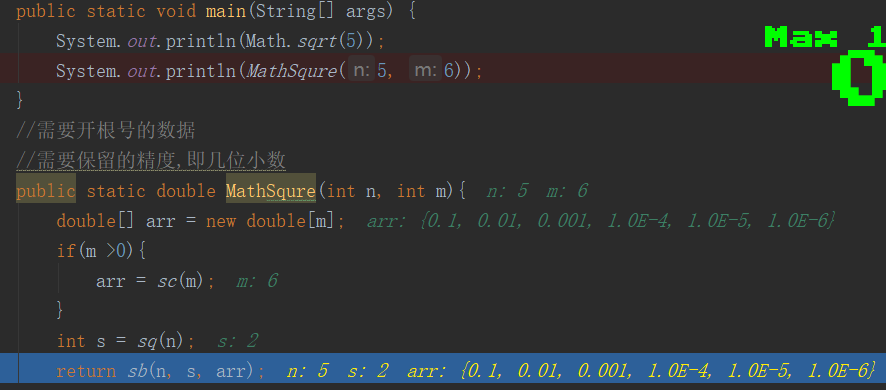来看代码

import java.math.BigDecimal;

/**
* @Auther: liuhaidong
* Data: 2020/4/21 0021、20:55
* Description:
* @version: 1.0
*/
public class Test6 {
public static void main(String[] args) {
System.out.println(Math.sqrt(5));
System.out.println(MathSqure(5, 6));
}
//需要开根号的数据
//需要保留的精度,即几位小数
public static double MathSqure(int n, int m){
double[] arr = new double[m];
if(m >0){
arr = sc(m);
}
int s = sq(n);
return sb(n, s, arr);
}
/**
* 计算要保留几位小数
* @param m
* @return
*/
public static double[] sc(int m){
double[] arr = new double[m];
int num = 0;
while(num != m){
double f = 1;
for(int i=0;i<=num;i++){
f = f*10;
}
arr[num] = 1/f;
num++;
}
return arr;
}
/**
* 计算整数位
* @param n
* @return
*/
public static int sq(int n){
if( n == 1){
return 1;
}
int tmp = 0;
for(int i=1;i<=n/2+1;i++){
if(i*i == n){
tmp = i;
break;
}
if(i*i > n){
tmp = i-1;
break;
}
}
return tmp;
}

/**
* 开根号
* @param n
* @param j
* @param arr
* @return
*/
public static double sb(int n, double j, double[] arr){
double tmp = j;
for(int p=0;p<arr.length;p++){
if(p>0){
j = tmp;//计算过后的值（整数位+小数位的和，赋值给j，下面继续运算）
}
for(int i=1;i<=9;i++){//小数位只有九位{0.1,0.2,0.3,0.4,0.5,0.6,0.7,0.8,0.9}
tmp = i*arr[p]+j;//i*arr[p],相当于每次加0.1,0.2 ...
if(tmp*tmp == n){
return tmp;
}
if(tmp*tmp >n){
//避免丢失精度
BigDecimal c1 = new BigDecimal(Double.toString(tmp));
BigDecimal c2 = new BigDecimal(Double.toString(arr[p]));
tmp = c1.subtract(c2).doubleValue();
break;
}
}
}
return tmp;
}
}

思路继续讲解。

小于2.3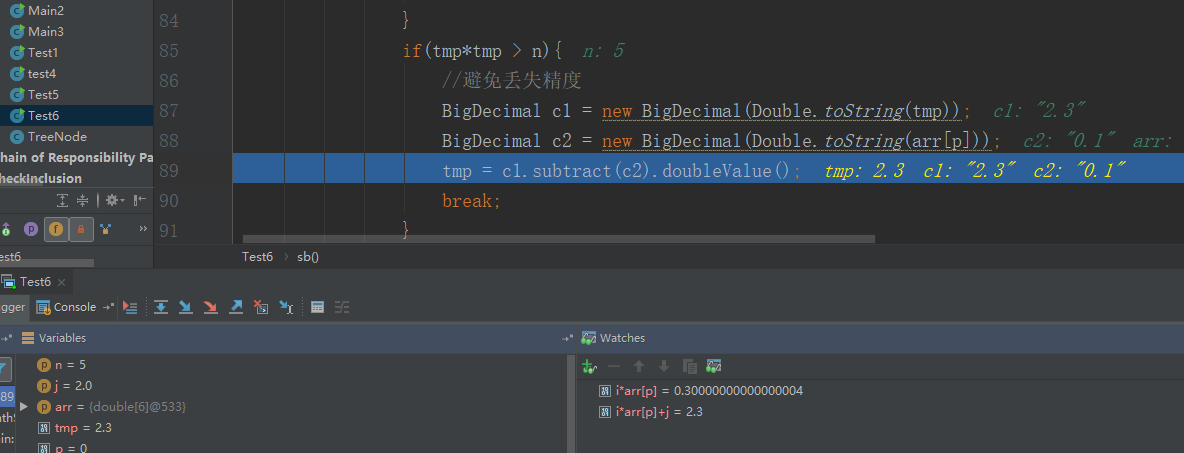把2.2记录在这里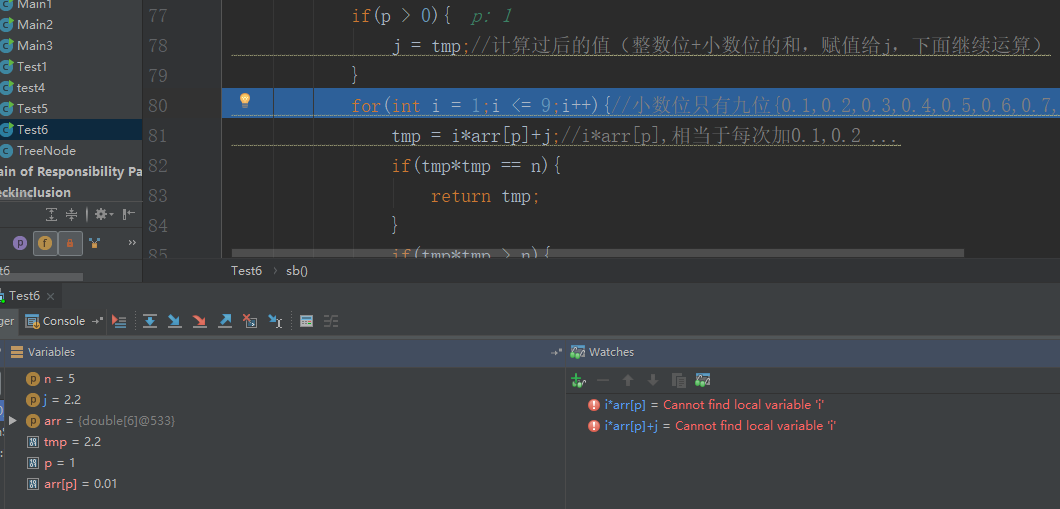小于2.24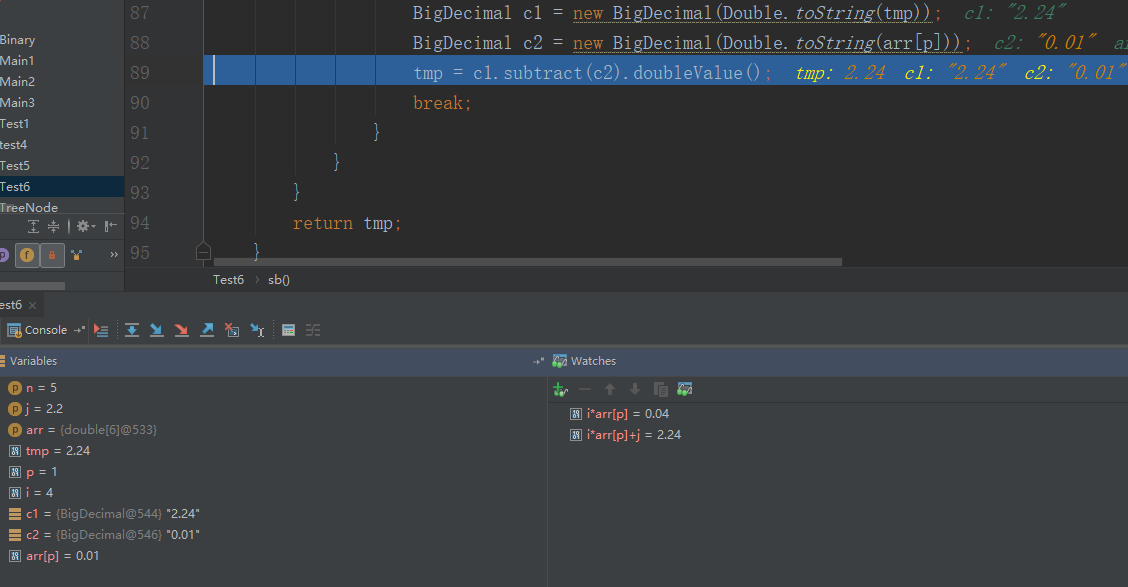记录2.23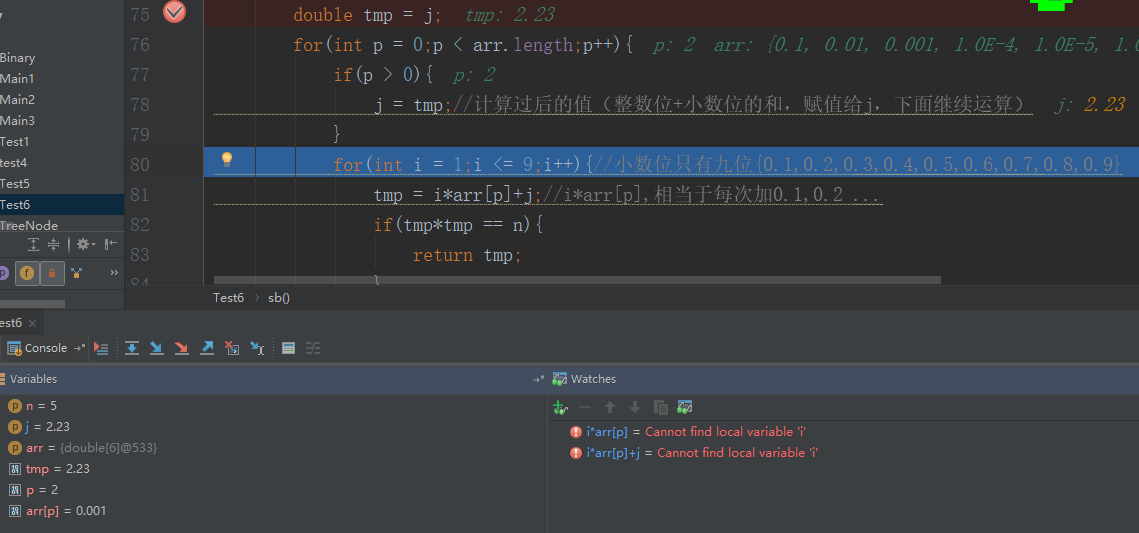依次这样做。本公众号分享自己从程序员小白到经历春招秋招斩获10几个offer的面试笔试经验，其中包括【Java】、【操作系统】、【计算机网络】、【设计模式】、【数据结构与算法】、【大厂面经】、【数据库】期待你加入！！！

展开全文开根号
• 使用Java自己实现开根号运算，网上也有不少代码，多数都使用String或者数组。这里写一段只使用double基础数据类型实现的方法。private static double sqrt(int n, int p) {double lower = 0;while (lower * lower &...

使用Java自己实现开根号运算，网上也有不少代码，多数都使用String或者数组。这里写一段只使用double基础数据类型实现的方法。

private static double sqrt(int n, int p) {

double lower = 0;

while (lower * lower < n) {

lower++;

}

--lower;

int flag = 1;

int d = 10;

while (flag <= p + 1) {

d = 10;

for (int x = 1; x < flag; x++) {

d = d * 10;

}

double step = 1.d / d;

for (int i = 0; i < 10; i++) {

if (lower * lower > n) {

break;

}

lower = lower + step;

}

lower = lower - step;

flag++;

}

double v1 = lower * (d * 10);

int v = ((int) v1 % 100) / 10;

double res;

if (v < 5) {

res = (lower * d - v) / d;

} else {

res = (lower * d - v + 10) / d;

}

return res;

}

参数中n是整数，p是精度。这种程序一般不能支持特别高的精度(比如几十位)，五六位就不错了。

代码原理简单，这里就不说了。先看一下输出：

public static void main(String[] args) {

System.out.println("2 1 = " + sqrt(2, 1));

System.out.println("2 2 = " + sqrt(2, 2));

System.out.println("2 3 = " + sqrt(2, 3));

System.out.println("2 4 = " + sqrt(2, 4));

System.out.println("3 2 = " + sqrt(3, 2));

System.out.println("3 3 = " + sqrt(3, 3));

System.out.println("25 4 = " + sqrt(25, 4));

System.out.println("25 6 = " + sqrt(25, 6));

}

输出

2 1 = 1.4000000000000004

2 2 = 1.41

2 3 = 1.414

2 4 = 1.4142

3 2 = 1.7300000000000004

3 3 = 1.7320000000000004

25 4 = 5.0000099999999925

25 6 = 5.000000099999996

由于double精度的问题，输出并不完全满足要求，当需要几位精度的时候要截取前几位。

另外，当输入的整数比较大的时候，精度丢失也比较快：

2500 5 = 50.00000000000001

2500 6 = 49.999999500000015

25 6 = 5.000000099999996

25 7 = 4.999999949999996

可以看到给25开根号6位精度还行，给2500开的话，6位精度已经不能保证了。

上面的代码在while确定整数部分的时候可以优化，并不需要把所有小于平方根的数都过滤一遍。读者可以尝试一下。

展开全文java 开根号
• java 不用内置函数开根号

千次阅读 2016-08-20 16:47:22
如题，刚开始用循环直接求了根号，后来模拟了内置函数sqrt求取 主要使用二分法，均已经运行可以出结果 直接上代码： 第一个： package test; import java.util.Scanner; public class xy { public static ...
如题，刚开始用循环直接求了根号，后来模拟了内置函数sqrt求取

主要使用二分法，均已经运行可以出结果
直接上代码：
第一个：
package test;
import java.util.Scanner;
public class xy
{
public static void main(String[] args)
{
System.out.println("请输入您的整数");
Scanner sc=new Scanner(System.in);
double a=sc.nextInt();
double b=0;double c=a;
for(double i=(a+b)/2;;)//a大b小
{
if(((i i-a)>=-0.00001 && (ii-a)<=0.00001) ||((a-i i) <=0.00001 &&(a-ii)>=-0.00001) )//相差小过0.0001
{
System.out.println(a+"取根号的结果是"+i);
break;
}
else if((i i-a)>=0.00001) //i值大
{
c=i;
i=(b+c)/2;
}
else
{
b=i;
i=(b+c)/2;
}
}
}
}
第二个：
package test;
import java.util.Scanner;
public class test
{
public static void main(String[] args)
{
System.out.println("输入你的要求取的值和次方数");
Scanner sc=new Scanner(System.in);
double shu=sc.nextDouble();//值
int a=sc.nextInt();//几次方
double temp=shu;
sqrt(shu,temp,a,0);
}
public static double power(double shu ,int ji)//求shu的ji次方
{
if(ji<=0)
return 1;
else if(ji==1)
return shu;
else
return shu
power(shu,ji-1);
}
public static double sqrt(double shu ,double temp,int ji,double a)
//a小数shu大数ji几次方
{
if(shu<0&&ji%2==0) { System.out.println("输入错误"); return 0; } else if(power((shu+a)/2,ji)-temp<=0.0000001 && power((shu+a)/2,ji)-temp>=-0.0000001)
{
System.out.println(temp+"开"+ji+"次方的值为"+(shu+a)/2);
return 1;
}
else if(power((shu+a)/2,ji)-temp>0.0000001)
{
shu=(shu+a)/2;
return sqrt(shu,temp,ji,a);
}
else
{
a=(shu+a)/2;
return sqrt(shu,temp,ji,a);
}
}
}
展开全文java 算法 二分法
• 匿名用户1级2014-05-25 回答在java.lang包中有个public final Math类，类中函数如下static double abs(double a)返回 double 值的绝对值。static float abs(float a)返回 float 值的绝对值。static int abs(int a)...java 开根号函数
• package classifier; public class getSqrt { public static void main(String[] args){ double a = 15; System.out.println(Math.sqrt(a)); System.out.println(Math.floor(Math.sqr...
• 最受欢迎的Java面试问题之一是，“给定一个整数x，编写一个Java程序来找到它的平方根”。解决这个问题有很多方法。在本文中，让我们看看在Java中查找平方根和平方根的不同方法。最受欢迎的Java面试问题之一是，...java开根号函数
• java里实现开根号

千次阅读 2021-02-26 10:26:28
java里实现开根号[2021-02-03 08:57:13]简介:php去除nbsp的方法：首先创建一个PHP代码示例文件；然后通过“preg_replace("/(\s|\&nbsp\;|　|\xc2\xa0)/", " ", strip_tags(\$val));”方法去除所有nbsp即可。推荐...java 开三次根号
• Java实现开根号

千次阅读 2018-09-30 02:21:47
Java实现开根号 二分法 static float sqr(float x){ float max = x; float min = 0; float mid=(min+max)/2; while (true){ if (Math.abs(x-mid*mid)&amp;lt;0.002) return mid;...开根号
• java实现开根号

万次阅读 2017-08-22 20:46:51算法 java
• import java.util.LinkedList; import java.util.List; public class Calc { final public static String[] OPT_ADD = { "+", "add", "plus", "jia" }; final public static String[] OPT_SUB = { "-", "sub", ...
• Java-求根号n(示例代码)

千次阅读 2021-02-26 13:57:00
平方，开根号java中是很简单的，Math.sqrt(double n)或者 Math.pow(double a, double b)，求a的b次方。但是我们可以自己想想，这些方法到底是怎么实现的。就拿开根号来解释，它有两种方法，二分法和牛顿迭代法。...java求根号值
• 展开全部java中对于大数BigInteger,BigDecimal开根号没有提供函数，可以参考以下实现方法：import java.math.BigDecimal;import java.math.BigInteger;public class BigSquareRoot {final static BigInteger HUNDRED...
• 需求：在不借助系统库的情况下，编写一个函数，实现开根号的操作，并且保证一定的精度 代码采用了牛顿迭代法以及二分查找法两种方式并分别打印了他们的循环次数以比较优劣： /** * 实现一个函数，完成对v开根号的...
• 以上定义的方法是开根号的代码实现 public static void main(String[] args) {  System.out.println(Math.sqart(11111)); System.out.println(Mathe.squrt(11111)); System.out.println("程序没结束");...java 编程
• Java-求根号n

2020-12-23 22:20:04
平方，开根号java中是很简单的，Math.sqrt(double n)或者 Math.pow(double a, double b)，求a的b次方。但是我们可以自己想想，这些方法到底是怎么实现的。就拿开根号来解释，它有两种方法，二分法和牛顿迭代法。...java 根号x
• 7-6 jmu-Java-01入门-开根号

千次阅读 2019-11-24 22:47:00
使用逐步逼近法对给定数值x求开根号。 逐步逼近法说明：从0开始逐步累加步长值。 步长=0.0001，epsilon(误差)=0.0001 循环继续的条件： 平方值<x 且 |x-平方值| > epsilon 说明与参考 数值输出保留6位小数，...
• 使用逐步逼近法对给定数值x求开根号。 逐步逼近法说明：从0开始逐步累加步长值。 步长=0.0001，epsilon(误差)=0.0001 循环继续的条件： 平方值<x 且 |x-平方值| > epsilon ###说明与参考 数值输出保留6位小数...
• 实现一个函数, 完成 开根号 的操作, 方法签名如下. double sqrt(int v, double t) 要求: 不能调用系统库函数, 诸如 Math.sqrt(v) 之类的; 假设计算出的结果为 r, 要求满足如下条件, , ...
• import java.util.Scanner;...使用逐步逼近法对给定数值x求开根号。 逐步逼近法说明：从0开始逐步累加步长值。 步长=0.0001，epsilon(误差)=0.0001 循环继续的条件： 平方值<x 且 |x-平方值| > epsilon ...java
• import java.util.*; public class Main{ public static void main(String[] args){ Scanner sc = new Scanner(System.in); while(sc.hasNext()){ double n = sc.nextDouble(); double e = 0.000001; double a =n; ...
• 1.0的平方根是：1.02.0的平方根是：1.4142150878906253.0的平方根是：1.73204803466796884.0的平方根是：2.0000019073486335.0的平方根是：2.23606872558593756.0的平方根是：2.44948959350585947.0的平方根是：2....java开根号函数
• 使用逐步逼近法对给定数值x求开根号。 逐步逼近法说明： 从0开始逐步累加步长值。 步长=0.0001，epsilon(误差)=0.0001 循环继续的条件： 平方值<x 且 |x-平方值| > epsilon ###说明与参考 数值输出保留6位小数...java
• 更多精彩内容，请关注公众号良许Linux，公众内回复1024可免费获得5T技术资料，包括：Linux，C/C++，Python，树莓派，嵌入式，Java，人工智能，等等。公众号内回复进群，邀请您进高手如云技术交流群。 公众号：良许...
• 今天我们来看一下这道题，java如何实现开根号的运算。大家都知道，java,c++,python等都有直接的函数可以给我们用，但是面试官这个时候让我们手撕源码，这个时候怎么做呢，来，不要慌！！！如果某个数字正好可以...
• java中sqrt函数的详解

万次阅读 2017-08-14 18:41:06
首先要明白，牛顿迭代法求的是函数和X轴的交点的横坐标，也就是我们说的根 1）那么第一步就是构建曲线了。 假设有一个数c，我们求它的平方根x，那么有一个等式，x^2 = c；挪到一边就是求 f = x^2 - c的根x 2）...sqrt java 平方根
• 小编典典安装JDK时，可以在内部找到标准库的源代码src.zip。...因此，它实际上只是一个本地调用，可能由Java在不同平台上以不同方式实现。但是，作为StrictMath状态文档：为了帮助确保Java程序的可移植...
• 递归与开根号运算

千次阅读 2018-12-18 20:06:51
# ...//涉及开根号最好用double以免数据缺失。 else if ( n == 1 ) sum = sqrt ( n + x ) ; return sum ; } //递归就使每一次循环对同一法则的使用并且有最后的退出条件。...

java开根号函数java 订阅